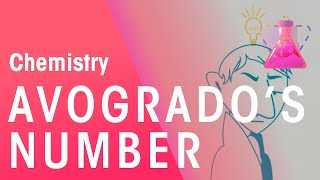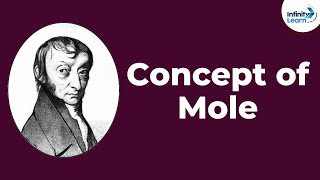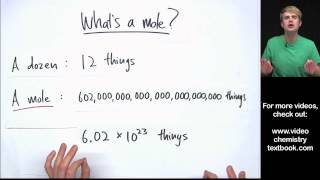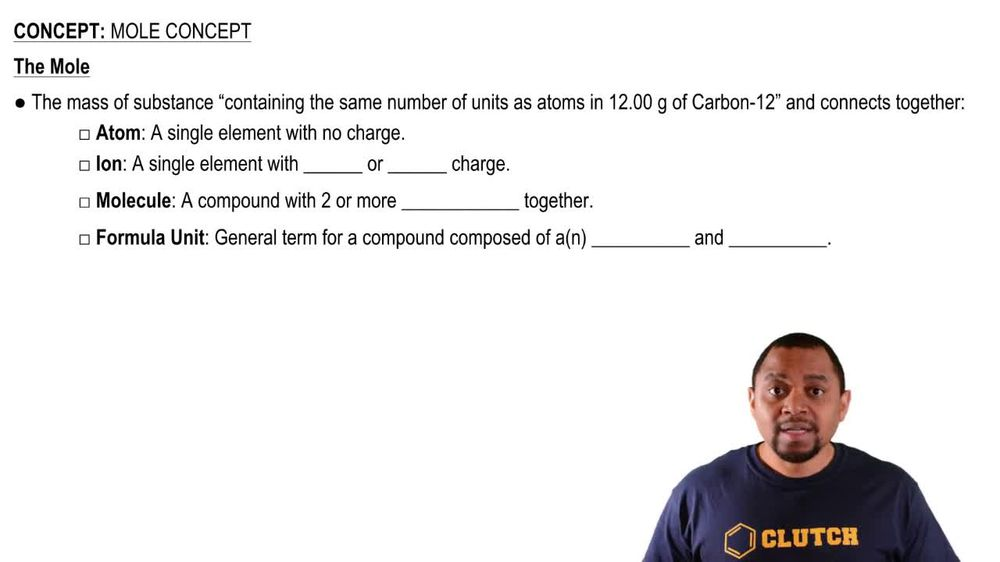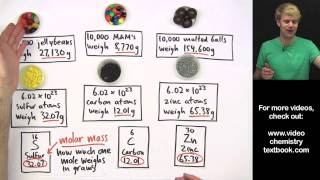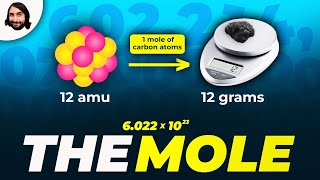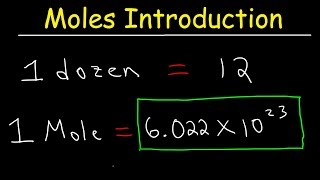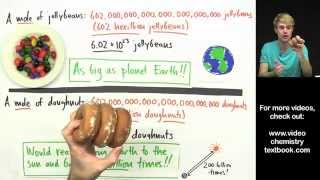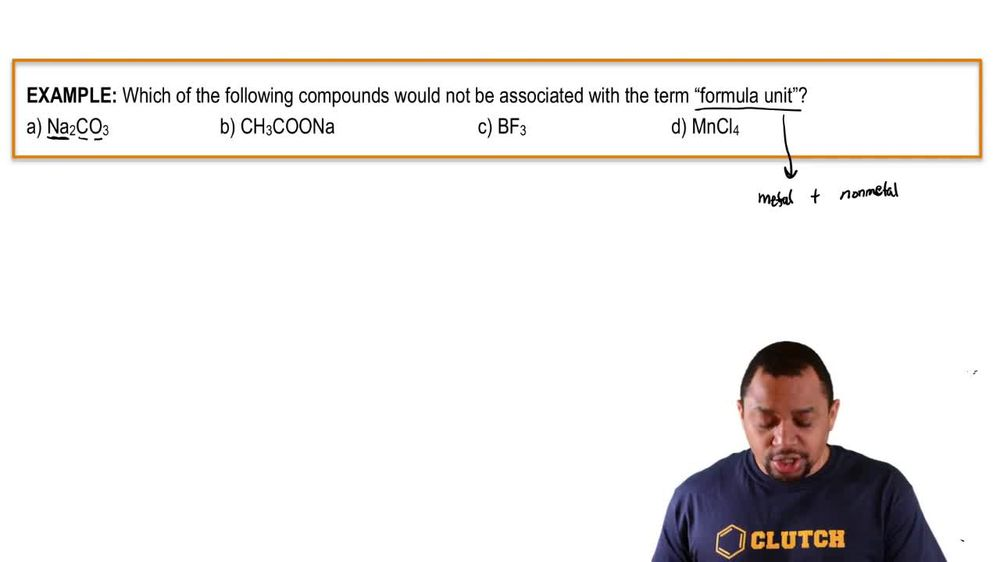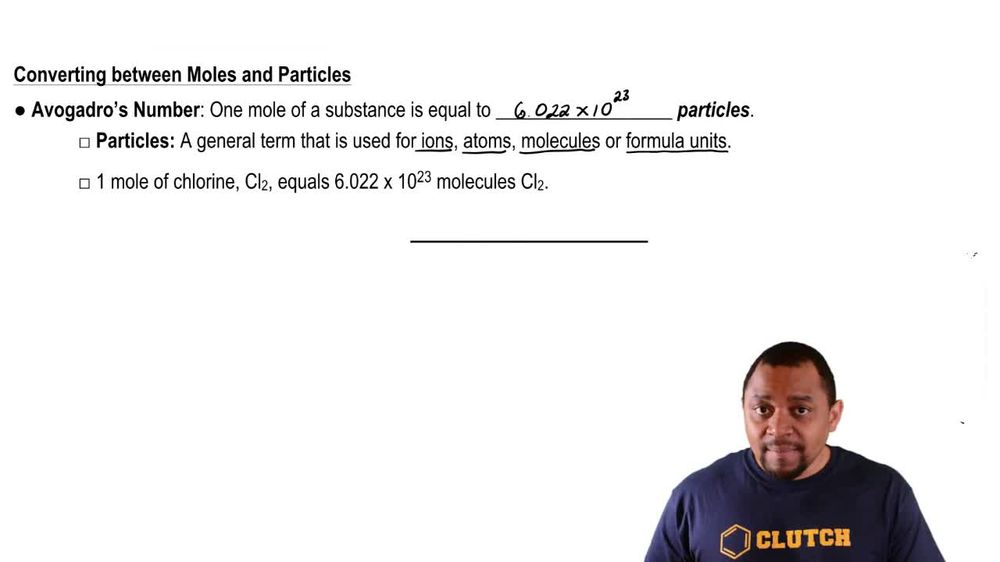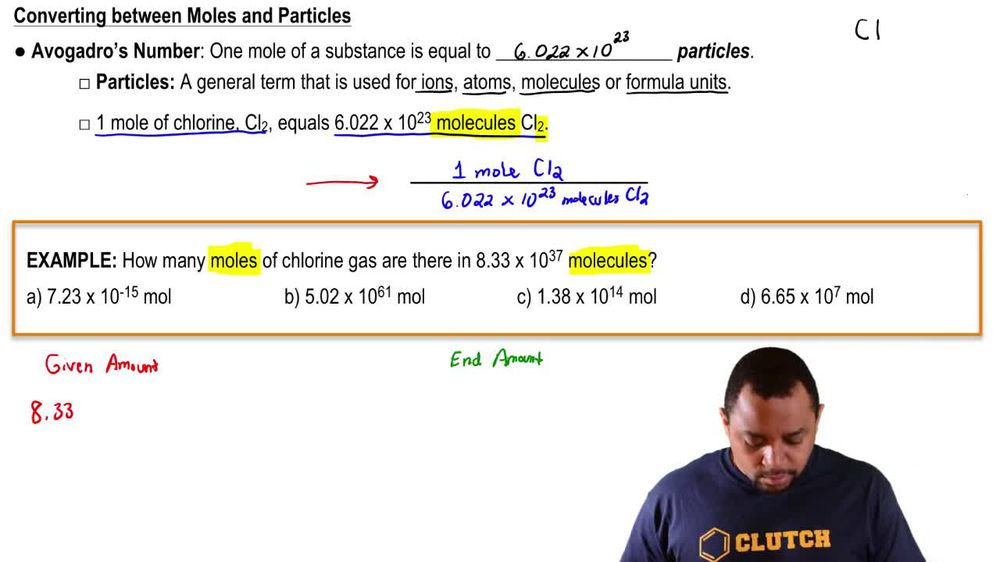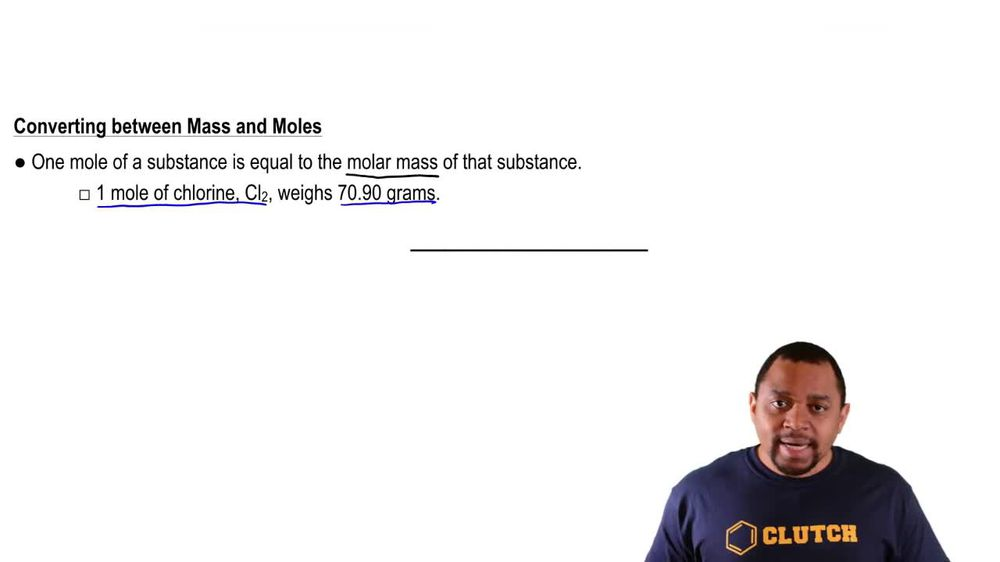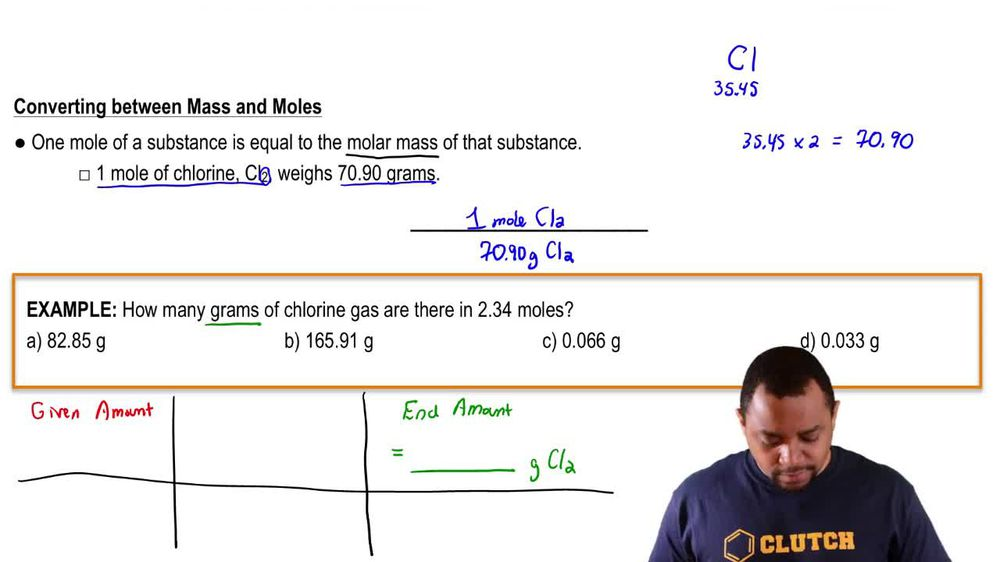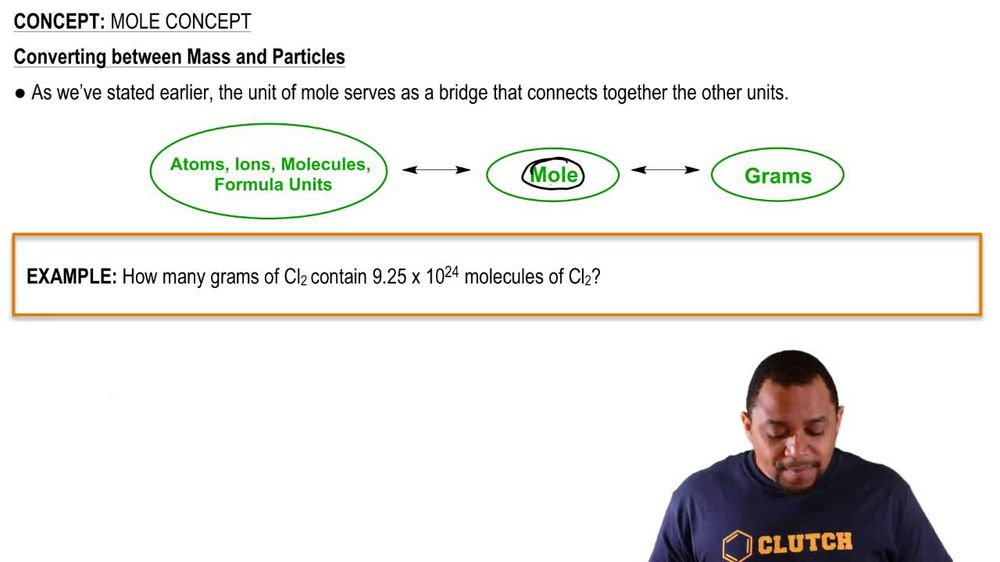Start typing, then use the up and down arrows to select an option from the list.
1. 2. Atoms & Elements2. Mole Concept
Problem

# Consider a sample of calcium carbonate in the form of a cube measuring 2.005 in. on each edge. If the sample has a density of 2.71 g>cm3, how many oxygen atoms does it contain?

Relevant Solution3m
Play a video:
Hi everyone here we have a question asking us to consider a sample of magnesium chloride cube that has an edge length of 10.0 centimeters. And our goal is to calculate the number of chlorine atoms in the cube. If its density is 2.32 g per centimeters cube. So the volume of a cube equals its side cubed. So in this case the volume of our cube Is going to equal 10 cm. Cute, Which equals 1000 centimeters. Cute. So now we need the molar mass of magnesium chloride. So we have magnesium and we have one of them. And if we look on the periodic table, its molar mass is 24. And then we have chlorine which we have two of and its molar mass is 35.45, which equals 70.9. And if we total that, we get 95.2 g per mole. So now to calculate the number of chlorine atoms, So we have centimeters cubed, Times 2.32 g purse centimeters cubed times one mole of magnesium chloride over its smaller mass. So 95 . g of magnesium chloride, Times two moles of chlorine because we have to multiple chlorine and magnesium chloride over one mole of magnesium. Cool ride times avocados number. So 6.22 times 10 to the 23rd Adams over one more of chlorine equals 2. Times 10 to the 25th Adams of corinne. And if we look at our dimensional analysis, we see that our centimeters cancel out our grands, cancel out our moles of magnesium chloride, cancel out our moles of chlorine, cancel out, and we're left with atoms, which is exactly what we want. So that is our final answer. Thank you for watching. Bye.